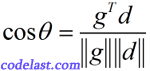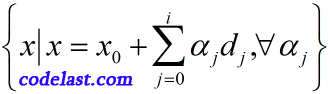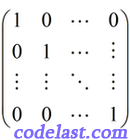# [原创] 再谈 共轭方向法/Conjugate Direction Method In Optimization

『1』向量共轭

$G$ 为对称正定矩阵，若 $d_m^TG{d_n} = 0,\;\;m \ne n$ ，则称 ${d_m}$${d_n}$ 为“G共轭”，共轭方向是“互不相关”的方向。

『2』特性

『3』理论基础

$g_{i + 1}^T{d_j} = 0,\;\;j = 0,1, \cdots ,i$$g_{i + 1}^T{d_j} = 0$ 这个式子中，当 $g$ 的下标是 ${i + 1}$ 时， $d$ 的下标可以是 $0,1,\cdots ,i$ ，例如， $g_3^T{d_0} = 0,\;\;g_3^T{d_1} = 0,\;\;g_3^T{d_2} = 0$ ，这表明，当前迭代点的梯度 ${g_3}$ 与前面所有的搜索方向（ ${d_0},{d_1},{d_2}$ ）直交。${x_0} + {\alpha _0}{d_0} + {\alpha _1}{d_1} = {x_1} + {\alpha _1}{d_1} = {x_2}$ ，所以这就说明了 ${x_1}$ 是在 ${{d_0}}$ 方向上line search得到的极小值点， ${x_2}$ 是在 ${{d_1}}$ 方向上line search得到的极小值点。所以由基础定理可知，当前迭代点的梯度与前面所有方向的点积为零。

『4』基本流程

• 确定一个搜索方向要满足： ${g_{k + 1}}^T{d_{k + 1}} < 0$ ——这是为了满足目标函数值下降的条件（下降是最优化的目标），并且 $d_{k + 1}^TG{d_i} = 0,\;\;i = 1,2, \cdots ,k$ ——这是为了满足搜索方向之间的“共轭”条件。
• 检验迭代终止条件，若未终止，则用line search求 $f({x_k} + {\alpha _k}{d_k}) = \mathop {\min }\limits_{\alpha \ge 0} f({x_k} + \alpha {d_k})$ ——在每一个搜索方向上，我们都要找到极小值点。
• ${x_{k + 1}} = {x_k} + {\alpha _k}{d_k}$ ，继续迭代

『5』创造共轭方向

Powell是谁？

M.J.D. POWELL，剑桥大学教授（已故），世界著名的最优化专家。他是袁亚湘的导师（袁亚湘，中国科学院数学与系统科学研究院研究员、博士生导师，美国数学学会首届会士（2012年），中国科学院院士）。

Powell方法是一种不需要求目标函数导数的方法（zero-order method）。有一篇英文文章里说，如果你只需要知道一种zero-order method如何编程实现的话，那么一定是选Powell方法，可见Powell方法是有其重要地位的。

『6』Powell方法的问题及改进
Powell方法产生的共轭方向集可能会变得线性相关，这会导致最终我们求得的，是N维空间的一个子空间内的极小值，而不是整体的极小值，所以，人们对Powell方法研究出了一些改进方案，例如：

• N轮迭代后，方向集重置为基向量；
• Brent（就是Brent's method的作者）提出，N轮迭代后，可以将方向集重置为任意正交矩阵（见下面的说明）的列向量；
• 放弃目标函数下降最大的方向，用一些好的方向代替N个必须共轭的方向；
• ...

PS：什么是正交矩阵➤➤ 版权声明 ➤➤### 《[原创] 再谈 共轭方向法/Conjugate Direction Method In Optimization》有2条评论

1. 共轭梯度迭代中的N怎么证明比最速下降的少呢？

回复
2. "所以由基础定理可知，当前迭代点的梯度与前面所有方向的点积为零"还是没有明白，求指点

回复